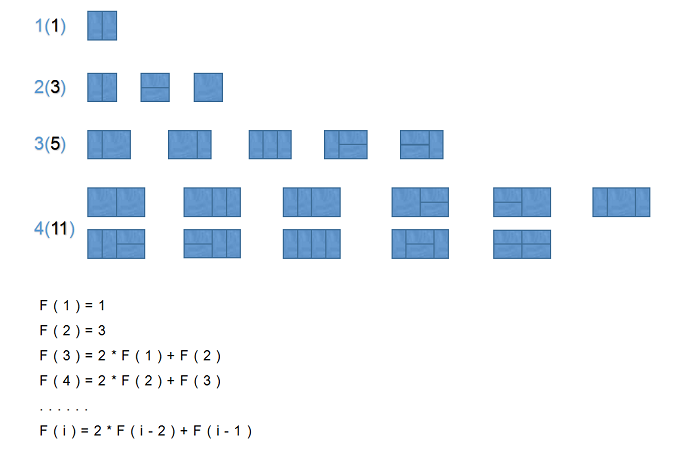Problem Description

Input

Output

Sample Input
3
2
8
12

Sample Output
3
171
2731

2个回答

`````` #include <stdio.h>
#include<math.h>
int function(int n) {
if (n == 1)
return 1;
if (n == 2)
return 3;
else
return 2 * function(n - 2) + function(n - 1);
}
int main() {
int n, tmp;
scanf("%d", &n);
for (int i = 0; i < n; i++) {
scanf("%d", &tmp);
printf("%d\n", function(tmp));
}
return 0;
}
``````